# Inelastic current in a silicon p-n junction¶

Version: 2017.2

Inelastic scattering effects due to electron-phonon interactions can play a fundamental role in determining the carrier transport through a device. In this tutorial, you will investigate the impact of such effects on the current through a silicon $$p$$-$$n$$ junction in reverse bias. In this system, carrier transport is dominated by band-to-band tunnelling between the valence band of the $$p$$ region and the conduction band of the $$n$$ region. This process can be enhanced considerably by inelastic scattering effects.

Specifically, you will use the InelasticTransmissionSpectrum module implemented in ATK to calculate the transmission spectrum of the device in the presence of electron-phonon interactions. You will also use the Inelastic Transmission Spectrum Analyzer plugin in QuantumATK to perform a detailed analysis of the current and determine which phonon mode is the main responsible for the current enhancement.

The methodology implemented in QuantumATK is based on the Lowest Order Expansion (LOE) [FPBJ07] and the eXtended LOE (XLOE) [LCF+14] methods. In this tutorial, you will use the XLOE method, which treats the finite energy difference between initial and final states in the transition $$\mathbf{k} \to \mathbf{k}\pm\mathbf{q}$$ due to electron-phonon coupling in a more accurate manner. However, the tutorial can be carried out also using the simpler LOE method, which will give qualitatively similar results. You can find more details about the theory underlying the two methods in the Notes sections of the InelasticTransmissionSpectrum module in the ATK manual.

## Creating the silicon p-n junction¶

To create the device, you can follow the instruction in the Create device section of the Silicon p-n junction tutorial. However, in the present tutorial you will create a shorter device to speed up the calculations. In theBuilder, contruct the device with the following changes:

• When using the Surface (Cleave) plugin to create a <100>-oriented structure, set the thickness to 24 layers instead of 52 layers;

• When using the Device from Bulk plugin, choose 5.4306 Å as the electrode length;

• Click the Doping plugin in Miscellaneous ‣ Doping to set the doping of the $$p$$ and $$n$$ regions. In this case, select the half of device to set the $$p$$ doping region and select the remainder part using the ctrl+i which will select the inversion part to set the $$n$$ doping region. In both regions, set a doping of $$2 \cdot 10^{19} \mathrm{e/cm^{3}}$$.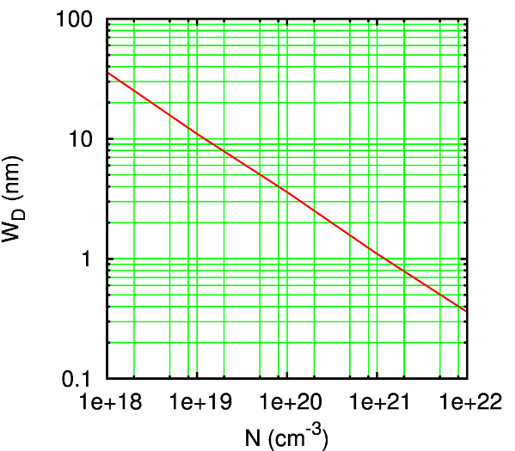You can download the resulting device configuration from here: Si_pn_junction.py.

## Transmission calculation without electron-phonon interactions¶

In this section, you will calculate and analyze the electronic structure of the $$p$$-$$n$$ junction at a reverse bias voltage of $$V_\mathrm{bias} = -0.4\ \mathrm{V}$$, and the associated transmission spectrum without electron-phonon interactions included, using the conventional Landauer formula as implemented in ATK in the TransmissionSpectrum module.

### Setting up the calculation¶

From theBuilder, send the structure to the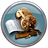Script generator using the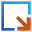button. Add the following blocks and set their parameters as follows:

Finally, in the Global IO options, change the name of the Default output file to transmission_V-0.4.hdf5.

Send the script to the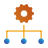Job manager using thebutton, save it as transmission_V-0.4.py and press thebutton to run the calculation. It will take less than 2 minutes on 4 CPUs. You can also download the full script from here: transmission_V-0.4.py.

### Analysis of the results¶

In the LabFloor, select theProjectedLocalDensityOfStates object from the file transmission_V-0.4.hdf5, and click on the Projected Local Density of States plugin. In the plugin window, select Spectral Current from the drop-down menu on the top left, and set the maximum value of the Data range to 0.1. In the panel on the left-hand side of the screen, use the Zoom button to make sure that the minimum value of the current is about $$10^{-24} \mathrm{A/eV}$$. The features below this value can be associated to numerical noise.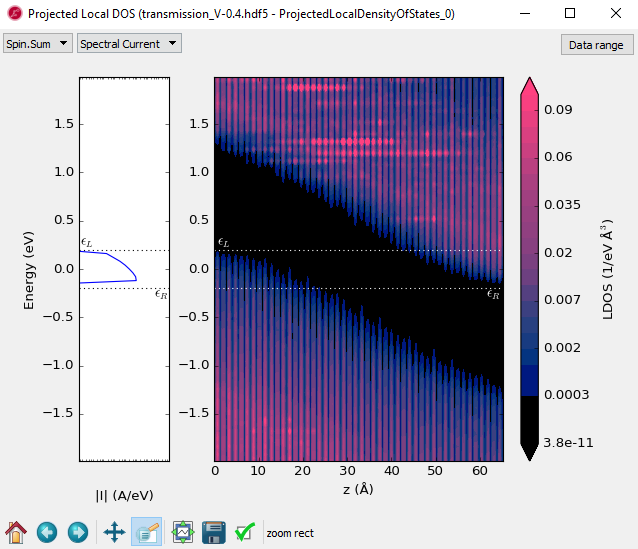From the left panel of the figure above, it can be seen that the maximum of the spectral current occurs inside the bias window ($$-0.2\ \mathrm{eV} \leq \mathrm{Energy} \leq 0.2\ \mathrm{eV}$$). Furthermore, from the right panel, which shows the density of states across the device, it can be seen that within the bias window, there are no electronic states in central region ($$20\ \mathrm{Å} \leq \mathrm{z} \leq 45\ \mathrm{Å}$$) of the device, so that tunnelling between the left and the right electrodes is the only possible transport mechanism. This indicates that electronic transport in the device in reverse bias is dominated by tunnelling.

To analyze the probability of tunnelling in $$\mathbf{k}$$-space, select theTransmission Spectrum object from the same file and click on the Transmission Analyzer plugin. In the plugin window, set the maximum value of the Data range to 0.1.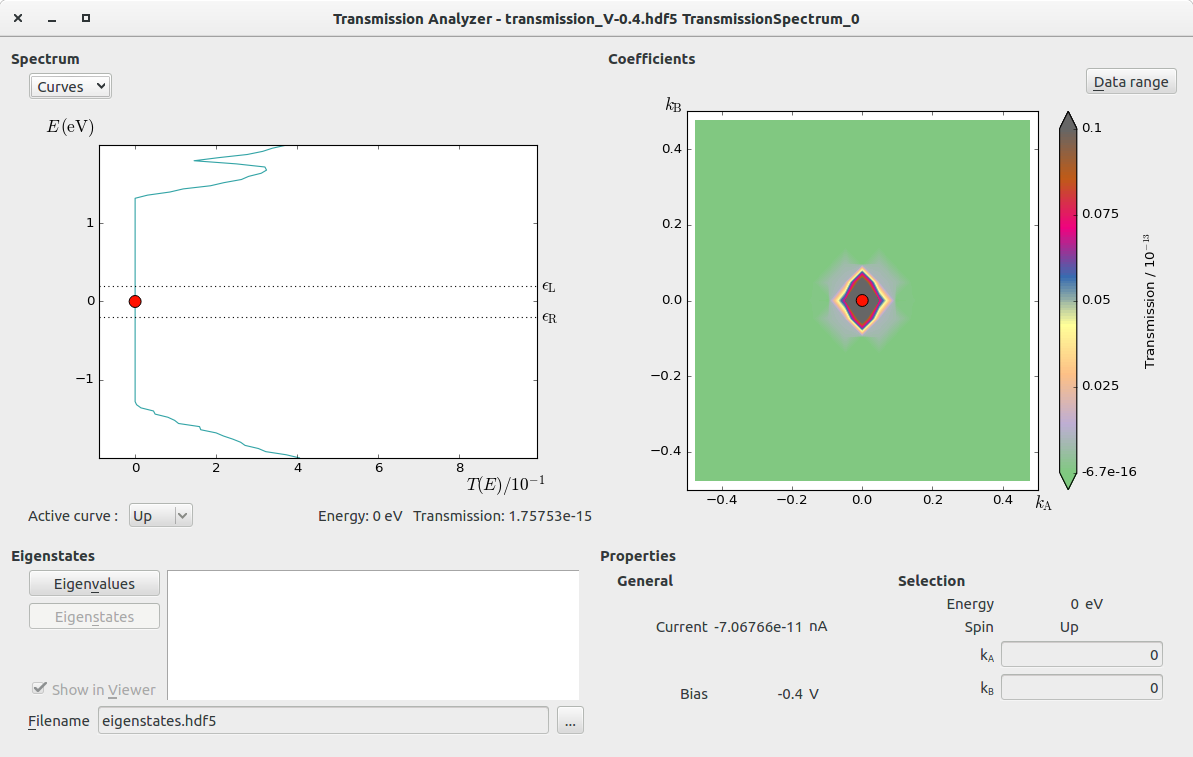From the figure above, it can be seen that the tunnelling probability is strongly peaked at the $$\Gamma$$-point of the Brilluoin zone at the Fermi level.

## Transmission calculation with electron-phonon interactions¶

In this section, you will calculate the transmission spectrum of the silicon $$p$$-$$n$$ junction at a reverse bias voltage of $$V_\mathrm{bias} = -0.4\ \mathrm{V}$$ including the effect of the electron-phonon interactions within the XLOE approximation [LCF+14].

To calculate the InelasticTransmissionSpectrum, you first need to calculate the DynamicalMatrix and the HamiltonianDerivatives of the system.

### Setting up the dynamical matrix calculation¶

In the LabFloor, drag and drop the DeviceConfiguration object contained in the file transmission_V-0.4.hdf5 to theScript generator. Remove the DeviceSemiEmpiricalCalculator and replace it with aForceFieldCalculator

• Select ‘StillingerWeber_Si_1985‘ from the list of available classical potentials in the Parameter set list.

Add the following blocks and set their parameters as follows:

Finally, in the Global IO options, change the name of the Default output file to dynmat.hdf5.

Send the script to theJob manager using thebutton, save it as dynmat.py and press thebutton to run the calculation. You can also download the full script from here: dynmat.py.

Note

Alternatively, you can also use the special thermal displacement - Landauer method (STD-Landauer) to include part of the electron-phonon coupling effects in the transmission calculation at a much lower computational cost. The STD-Landauer case study treats a system which is similar to that discussed in this tutorial.

### Setting up the Hamiltonian derivatives calculation¶

In the LabFloor, drag and drop the DeviceConfiguration object contained in the file transmission_V-0.4.hdf5 to theScript generator, and modify theNew Calculator block as follows:

• In Hamiltonian tick the ‘No SCF iteration‘ option.

Add an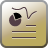Study Objects ‣ HamiltonianDerivatives block and set the parameters as follows:

• Set Repetitions to ‘Custom
• Set the number of repetitions to:
• $$\mathrm{n_A} = 3$$
• $$\mathrm{n_B} = 3$$
• $$\mathrm{n_C} = 1$$
• In Constraints, click Add and constrain the electrode repetitions with Fixed constrains. The resulting list of constrained atoms should be: 0,1,2,3,44,45,46,47.
• Untick the ‘Equivalent Bulk‘ option

In the Global IO options, change the name of the Default output file to dHdR_V-0.4.hdf5.

Send the script to theJob manager using thebutton, save it as dHdR_V-0.4.py and press thebutton to run the calculation. The calculation will take around 20 minutes on 8 CPUs. You can also download the full script from here: dHdR_V-0.4.py.

Note

Here, the Hamiltonian derivatives are calculated non self-consistently to speed up the calculations.

### Calculating the inelastic transmission spectrum¶

To calculate the inelastic part of the current, click theScript generator, and add the following blocks:

Open the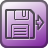Analysis from File block, select the transmission_V-0.4.hdf5 file and load the DeviceConfiguration object contained in the file .

You will notice that two additional blocks have also been added:

In our case, the dynamical matrix and the Hamiltonian derivatives have already been calculated previously and can be re-used. Delete the two blocks, add two moreAnalysis from File blocks and arrange them as shown in the figure below:Click on the secondAnalysis from File block from the top. Select the file dynmat.hdf5 and load the DynamicalMatrix object. Uncheck the DeviceConfiguration object included in the same file. The panel should look like as in the figure below:Then, click on the thirdAnalysis from File block from the top. Select the file dHdR_V-0.4.hdf5 and load the HamiltonianDerivatives object. The panel should look like as in the figure below:Finally, set the parameters for theAnalysis ‣ InelasticTransmissionSpectrum block as shown below:

• In the Energy part, set:

• $$\mathrm{E_0} = -0.5\ \mathrm{eV}$$
• $$\mathrm{E_1} = 0.5\ \mathrm{eV}$$
• Points = 25
• In the k-point Sampling part:

• set Grid type to ‘Regular k-point grid

• set the grid range for $$\mathrm{k_A}$$ and $$\mathrm{k_B}$$ to $$[-0.2 : 0.2]$$

• set the Sampling to:

• $$\mathrm{k_A} = 3$$
• $$\mathrm{k_B} = 3$$
• In the q-point Sampling part:

• set Grid type to ‘Regular q-point grid

• set the grid range for $$\mathrm{q_A}$$ and $$\mathrm{q_B}$$ to $$[-0.5 : 0.5]$$

• set the Sampling to:

• $$\mathrm{q_A} = 9$$
• $$\mathrm{q_B} = 9$$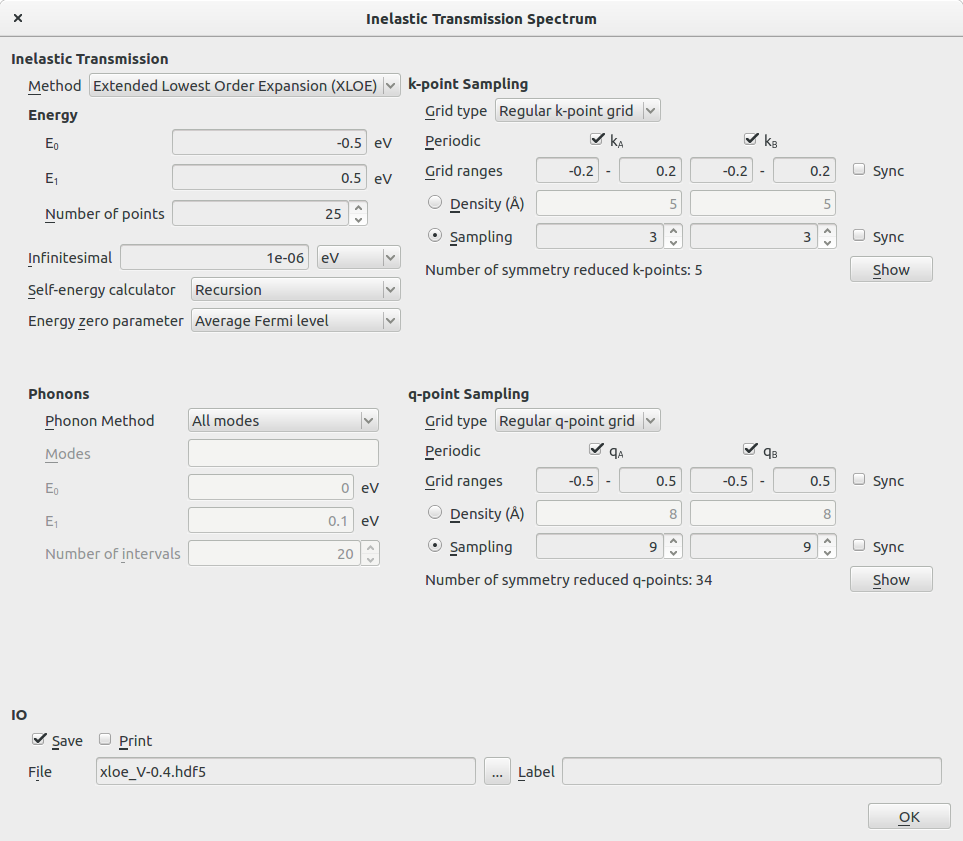In the Global IO options, change the name of the Default output file to xloe_V-0.4.hdf5.

Send the script to theJob manager using thebutton, save it as xloe_V-0.4.py and press thebutton to run the calculation. The calculation will take around 1.5 hour on 24 CPUs. You can also download the full script from here: xloe_V-0.4.py.

### Analysis of the inelastic transmission spectrum¶

The results of the inelastic transmission spectrum can be analyzed in detail using the Inelastic Transmission Spectrum Analyzer.

In the LabFloor, select theInelasticTransmissionSpectrum object contained in the file xloe_V-0.4.hdf5, and click on the Inelastic Transmission Spectrum Analyzer plugin in the plugins panel on the right-hand side of the screen.

First of all, you will analyze the $$\mathbf{k}$$- and $$\mathbf{q}$$-dependency of the current. In the main window of the analyzer, set the following parameters:

• Plot type : ‘Current vs. k-points
• Bias voltage : $$-0.4\ \mathrm{V}$$

You will obtain a figure similar to the one below, showing the $$\mathbf{k}$$-dependency of the current within the sampled range of k-points. From the figure, it is evident that the main contribution to the current comes from the $$\Gamma$$-point of the two-dimensional Brillouin zone defined by $$k_\mathrm{A}$$ and $$k_\mathrm{B}$$, with a non-negligible contribution from finite $$\mathbf{k}$$-vectors.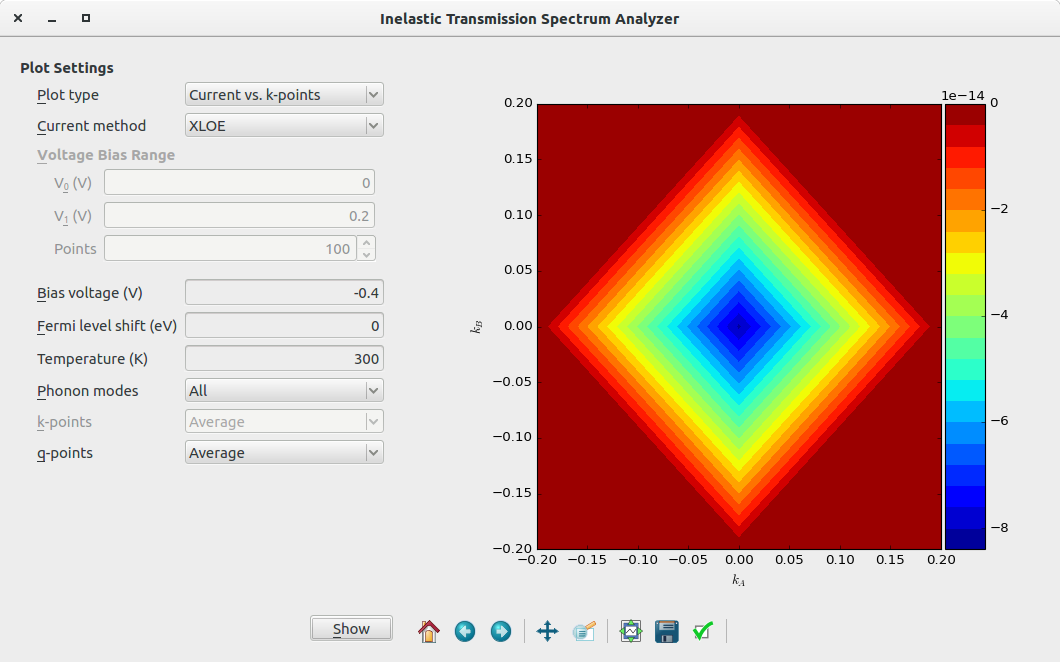Next, change the Plot type to ‘Current vs. q-points‘. You will obtain a figure similar to the one below, showing the $$\mathbf{q}$$-dependency of the current. From the figure, it is evident that also in this case the main contribution to the current comes from the $$\Gamma$$-point of the two-dimensional Brillouin zone defined by $$q_\mathrm{A}$$ and $$q_\mathrm{B}$$, , with a non-negligible contribution from finite $$\mathbf{q}$$-vectors.The two figures above indicate that the current is mainly associated with transitions occurring at the $$\Gamma$$-point of the two-dimensional Brillouin zone, both in $$\mathbf{k}$$-space and in $$\mathbf{q}$$-space. To analyze which of the phonon modes at the $$\Gamma$$-point is the one that contributes the most, set the following parameters in the main window of the analyzer:

• Plot type : ‘Current vs. phonon mode

• k-points : ‘(0.00, 0.00)

• q-points : ‘(0.00, 0.00)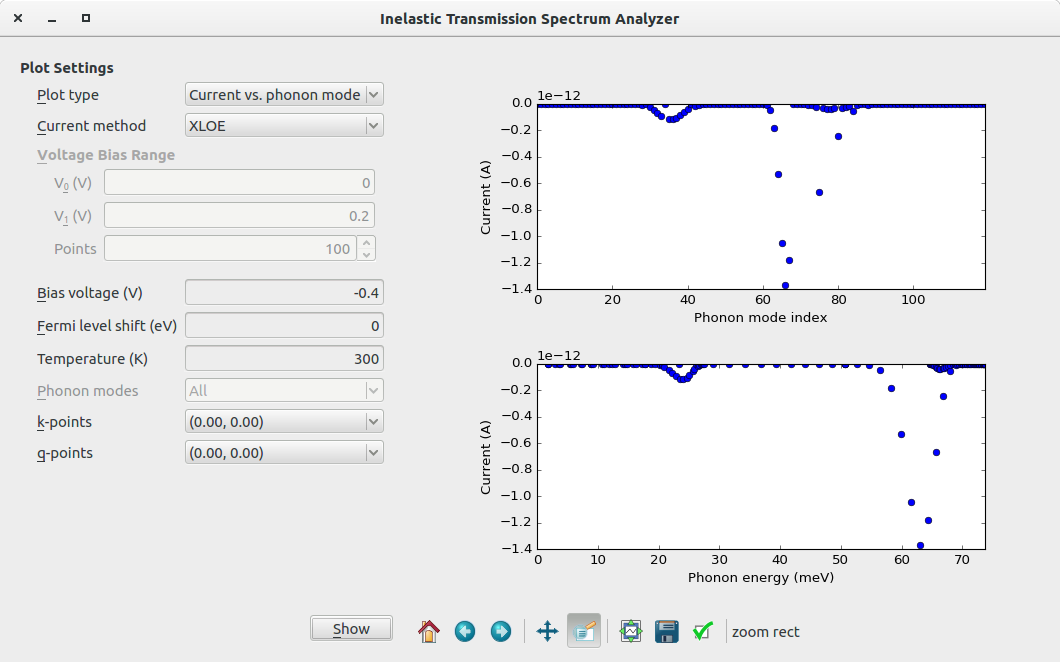From the figure, it can be seen that the phonon that contributes the most to the current is that with index $$66$$, and energy $$\hbar \omega = 63.01\ \mathrm{meV}$$.

This phonon mode can be visualized by selecting the VibrationalMode object in the file dynmat.hdf5 calculated in Section Setting up the dynamical matrix calculation and using the Vibration Visualizer plugin: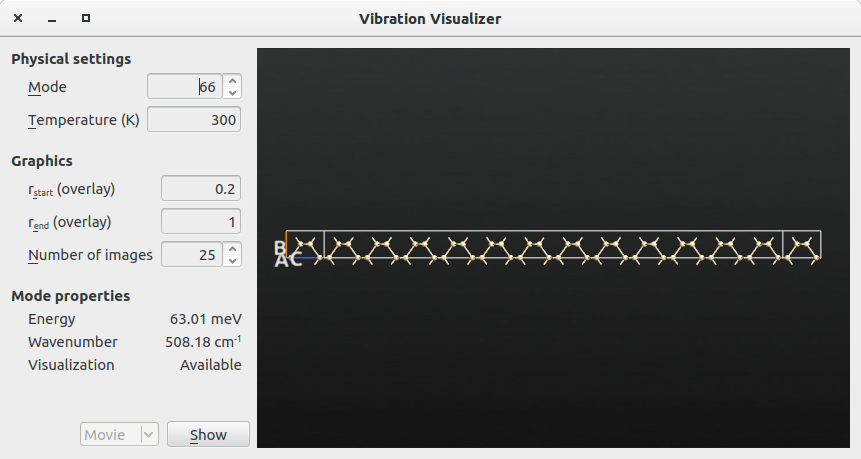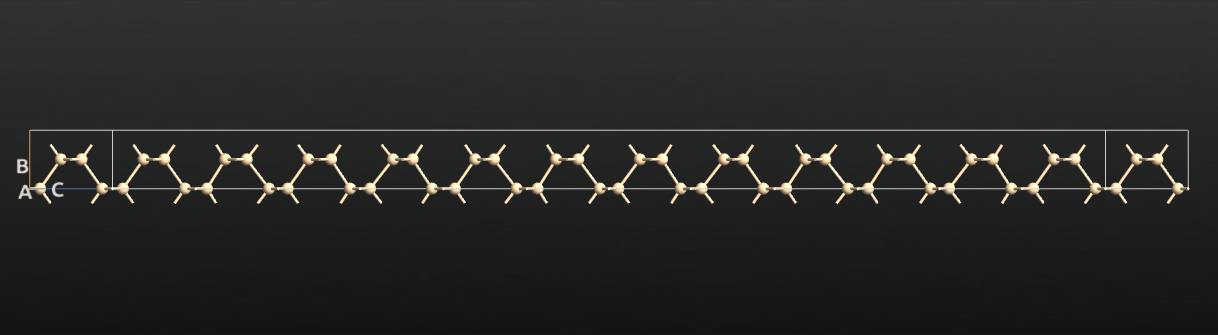Note

In the figures presented above, the values of the current are negative, because the simulations are performed in reverse bias conditions.

### Calculation of the current with and without electron-phonon interactions¶

In order to calculate the current without and with electron-phonon interactions, download the script current.py and run it from the terminal as:

atkpython current.py transmission_V-0.4.hdf5 xloe_V-0.4.hdf5.

Note

On windows, it is not convenient to run current.py script from the terminal. In this script, you can replace filename1 and filename2 to the transmission_V-0.4.hdf5 and xloe-V-0.4.hdf5. In order to run it, drag it on theJob manager.

The script will calculate the elastic ($$I_\mathrm{el}$$) and inelastic ($$I_\mathrm{inel}$$) components of the current. The total current without and with electron-phonon interactions will then be calculated as:

$I (\mathrm{w/o\ interactions}) = I_\mathrm{el}$$I (\mathrm{w\ interactions}) = I_\mathrm{el} + I_\mathrm{inel}$

The output of the calculation will show the name of the files used as input, as well as the values of the calculated currents (highlighted in yellow):

 +------------------------------------------------------------------------------+
|                                                                              |
| QuantumATK 2017.2 [Build 997d195]                                     |
|                                                                              |
+------------------------------------------------------------------------------+
--------------------------------------------------------------------------------
TransmissionSpectrum read from file :
transmission_V-0.4.hdf5
InelasticTransmissionSpectrum read from file :
xloe_V-0.4.hdf5
--------------------------------------------------------------------------------
Current (w/o interactions)    = -3.71e-09 nA
Current (w interactions)      = -9.03e-06 nA
--------------------------------------------------------------------------------

Timing:                          Total     Per Step        %

--------------------------------------------------------------------------------

Loading Modules + MPI   :       1.33 s       1.33 s       2.58% |=============|
--------------------------------------------------------------------------------
Total                   :      51.58 s


Note

The above calculated currents are different with the currents before analysis of the InelasticTransmissionSpectrum because of different k-points samplings. Also in this case, the value of the current is negative, because the simulations are performed in reverse bias conditions.

It can be seen that electron-phonon scattering leads to an increase in the reverse bias current of about three orders of magnitude!

## Speeding up the calculations¶

ATK implements several methods which can be used to speed up the calculations considerably and enable the calculation of the inelastic transmission for devices comprised of thousands of atoms. These methods are:

For a more detailed description of these methods, see the section Large Device Calculations of the InelasticTransmissionSpectrum module in the ATK manual.

### The ‘Phonon energy intervals’ method¶

This method allows us to group $$3N$$ phonon modes of a device with $$N$$ vibrating atoms into $$M$$ energy intervals to form $$M$$ new effective phonon modes, with $$M << 3N$$. The inelastic transmission spectrum will therefore be calculated only for these $$M$$ effective phonon modes, greatly reducing the computational cost of the calculation.

In the LabFloor, drag and drop the DeviceConfiguration object contained in the file transmission_V-0.4.hdf5 to theScript generator. Setup the calculation of the inelastic transmission spectrum as in the Calculating the inelastic transmission spectrum Section, but in this case set the Phonon Method in the Phonons part to ‘Phonon energy intervals‘.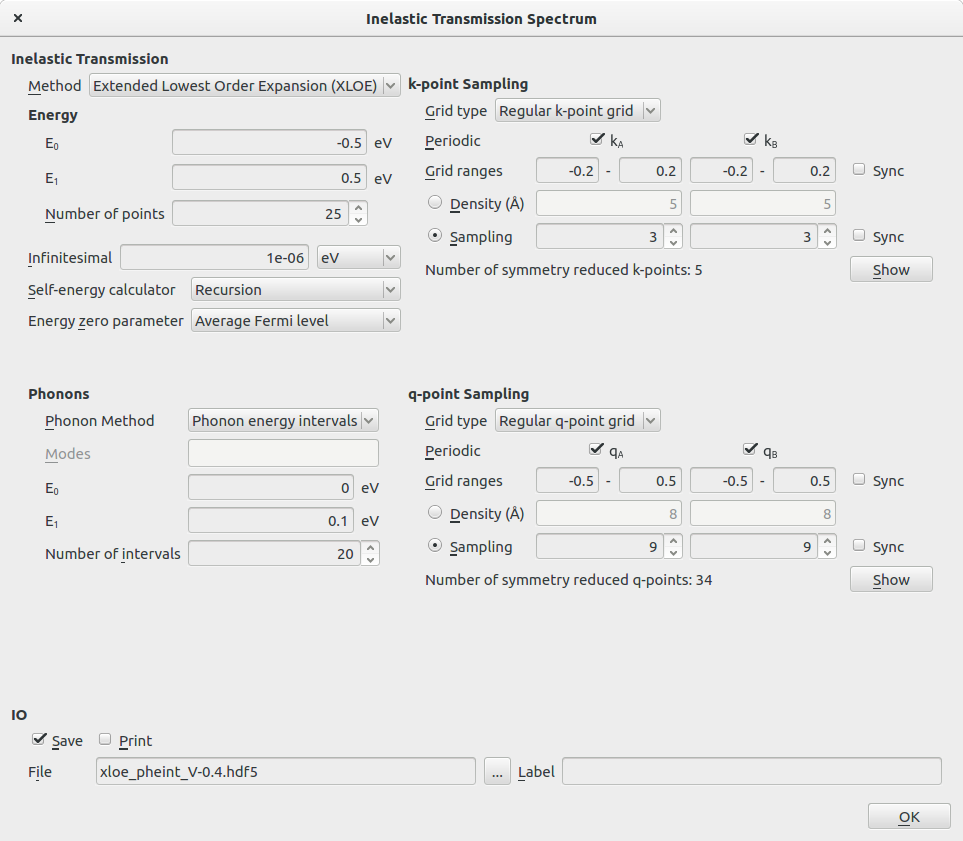Note

The default parameters of the Phonon energy intervals are fine for the present calculation, but in general one should ensure that the energy range $$[E_\mathrm{0}:E_\mathrm{1}]$$ spans that of the phonon modes of the calculated dynamical matrix.

In the Global IO options, change the name of the Default output file to xloe_pheint_V-0.4.hdf5.

Send the script to theJob manager using thebutton, save it as xloe_pheint_V-0.4.py and press thebutton to run the calculation. The calculation will take only 20 minutes on 24 CPUs. You can also download the full script from here: xloe_pheint_V-0.4.py.

Once the calculation is finished, run the script current.py as:

atkpython current.py transmission_V-0.4.hdf5 xloe_pheint_V-0.4.hdf5.

If you are a Windows user, see how to run the script.

The resulting output will be:

 +------------------------------------------------------------------------------+
|                                                                              |
| QuantumATK 2017.2 [Build 997d195]                                     |
|                                                                              |
+------------------------------------------------------------------------------+
--------------------------------------------------------------------------------
TransmissionSpectrum read from file :
transmission_V-0.4.hdf5
InelasticTransmissionSpectrum read from file :
xloe_pheint_V-0.4.hdf5
--------------------------------------------------------------------------------
Current (w/o interactions)    = -3.71e-09 nA
Current (w interactions)      = -9.25e-06 nA
--------------------------------------------------------------------------------

Timing:                          Total     Per Step        %

--------------------------------------------------------------------------------

Loading Modules + MPI   :       1.34 s       1.34 s      19.27% |=============|
--------------------------------------------------------------------------------
Total                   :       6.94 s


One can see that the calculated values for the current are essentially the same as those calculated in the Section Calculation of the current with and without electron-phonon interactions, despite the fact that the present calculations are much faster.

### Using the bulk dynamical matrix and Hamiltonian derivatives¶

Another option to speed up the calculations is to use the dynamical matrix and Hamiltonian derivatives of the bulk electrodes, instead of those of the device. However, this is possible only for devices with a structure which is translationally invariant along the $$\mathrm{C}$$-direction, apart from doping, electrostatic regions and applied bias voltage.

The scripts needed to calculate the dynamical matrix and the Hamiltonian derivatives of the bulk electrodes can be downloaded from here: dynmat_bulk.py, dHdR_bulk.py. Running the two calculations on 4 CPUs will take less than 2 minutes.

In order to calculate the current inelastic transmission spectrum using the bulk dynamical matrix and Hamiltonian derivatives, repeat the steps followed in Section Calculating the inelastic transmission spectrum, with the changes described in the following.

First, click theScript generator, add the following blocks, and modify their parameters as follows:

Remove theDynamicalMatrix andHamiltonianDerivatives blocks, add two moreAnalysis from File blocks right after theAnalysis from File already present, and set their properties as follows:

dynamic_bulk.hdf5 file and load the DynamicalMatrix object contained in the file.

dHdR_bulk.hdf5 file and load the HamiltonianDerivatives object contained in the file.

In the Global IO options, set the name of the Default output file to xloe_V-0.4_bulk.hdf5. In this case, you need to modify the Inelastic Transmission Spectrum block in the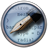Editor as follows:

  1 2 3 4 5 6 7 8 9 10 11 12 13 14 inelastic_transmission_spectrum = InelasticTransmissionSpectrum( configuration=device_configuration, bulk_dynamical_matrix=dynamical_matrix, bulk_hamiltonian_derivatives=hamiltonian_derivatives, energies=numpy.linspace(-0.5, 0.5, 25)*eV, kpoints=kpoints, qpoints=qpoints, self_energy_calculator=RecursionSelfEnergy(), energy_zero_parameter=AverageFermiLevel, infinitesimal=1e-06*eV, phonon_modes=All, method=XLOE, spectral_representation=True, electrode_extensions=[0, 0], 

Send the script to theJob manager using thebutton, save it as xloe_V-0.4_bulk.py and press thebutton to run the calculation. Also in this case, the calculation will take only 20 minutes on 24 CPUs. You can also download the full script from here: xloe_V-0.4_bulk.py.

Once the calculation is finished, run the script current.py as:

atkpython current.py transmission_V-0.4.hdf5 xloe_V-0.4_bulk.hdf5.

If you use Windows, look at the how to run the script.

The resulting output will be:

 +------------------------------------------------------------------------------+
|                                                                              |
| QuantumATK 2017.2 [Build 997d195]                                     |
|                                                                              |
+------------------------------------------------------------------------------+
--------------------------------------------------------------------------------
TransmissionSpectrum read from file :
transmission_V-0.4.hdf5
InelasticTransmissionSpectrum read from file :
xloe_V-0.4_bulk.hdf5
--------------------------------------------------------------------------------
Current (w/o interactions)    = -3.71e-09 nA
Current (w interactions)      = -6.42e-06 nA
--------------------------------------------------------------------------------

Timing:                          Total     Per Step        %

--------------------------------------------------------------------------------

Loading Modules + MPI   :       1.33 s       1.33 s       2.17% |=============|
--------------------------------------------------------------------------------
Total                   :      61.15 s (1m01.15s)


It can be seen that the calculated values for the current is similar to that calculated using the dynamical matrix and Hamiltonian derivatives of the device configuration, although there are differences due to the fact that in the present case, the loss translational invariance of the Hamiltonian derivatives due to the applied bias is not taken into account.